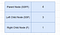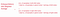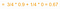# Decision Trees in Machine Learning (ML) with Python Tutorial

## Diving into decision trees in machine learning (ML) with Python

Last updated, January 8, 2021

Author(s): Saniya Parveez, Roberto Iriondo

This tutorial’s code is available on Github and its full implementation as well on Google Colab.

📚 Check out our gradient descent tutorial. 📚

# What is a Decision Tree?

A decision tree is a vital and popular tool for classification and prediction problems in machine learning, statistics, data mining, and machine learning . It describes rules that can be interpreted by humans and applied in a knowledge system such as databases. Fundamentally, a decision tree T encodes d (a classifier or regression function) in the form of a tree structure which presents the following attributes:

• Decision node: It defines a test on a single attribute.
• Leaf node: It shows the value of the target attribute.
• Edge: It is a split of one attribute.
• Path: It is a disjunction of the test to make the final decision.

These are other names that decision trees are known as:

• Tree classifier.
• Divide and conquer strategy.
• Hierarchical classifier.
• Multistage classifier.

It classifies cases by commencing at the tree’s root and passing through it unto a leaf node.

A decision tree uses nodes and leaves to make a decision.

Representation of the classification above in the form of a decision tree:

# Decision Tree Example

## Problem Statement

Predict the result of the basketball game between two different teams — team1 and team2.

## List of Usable Knowledge or Attributes related to the Game

• Did Peter play center or forward?
• What was the location of the game — home or away?
• What was the start time of the game?
• Was the opponent’s center tall or not?

## Data for Prediction

Therefore, based on figure 5, historical and prediction data:

• Generalize the learned rule to new data.
• It is a classification problem.

# Building a Decision Tree

Decision tree learning involves learning a sequence of if/else queries that get us to the “true” answer almost immediately. These questions are also called test. It searches over all possible tests and finds the one that is most instructive about the target variable.

## How to grow a decision tree?

• The top node is also called the root, and it represents the whole dataset.
• A decision tree progressively splits the training set into smaller and smaller subsets.
• If the test is “true” — a point is assigned to the left node; otherwise, it is assigned to the right node.
• A leaf of a tree that contains data points and it shares the same target values is called pure .
• A prediction on a new data point is made by examining which region of the partition of the feature space the point lazes in and then predicting the majority target in that region .
`from sklearn.tree import DecisionTreeClassifierfrom sklearn.datasets import load_breast_cancerfrom sklearn.model_selection import train_test_splitcancer = load_breast_cancer()X_train, X_test, y_train, y_test = train_test_split(cancer.data, cancer.target, stratify=cancer.target, random_state=42)`
• If we do not restrict a decision tree's depth, it can become arbitrarily deep and complex.
• As shown in figure 6, the accuracy on the training set is 100% — because the leaves are pure, the tree has grown deep enough to remember all the labels on the training data unquestionably.
• The tree can grow vast and such kinds of trees are hard to understand. Larger trees are typically less accurate than smaller trees .

Code implementation to create leaf of a decision tree (The leaf is created based on the ml_tasks — regression or classification):

`def create_leaf(data, ml_task):    label_column = data[:, -1]        if ml_task == "regression":        leaf = np.mean(label_column)    else:        unique_classes, counts_unique_classes = np.unique(label_column, return_counts=True)        i = counts_unique_classes.argmax()        leaf = unique_classes[i]        return leaf`

The main aim of a decision tree is to select appropriate features for splitting the tree into subparts. Then we apply an ID3 algorithm in the background during splitting.

# Node Impurity

Node impurity is the homogeneity within a node. A node is impure if cases have more than one value for the response. A node is pure if all instances have the same value for the response or target variable or impurity = 0.

These are the two most popular methods for measuring node impurity:

• Entropy.
• Gini.

The best split is selected based on the degree of impurity of the child nodes. Node impurity is 0 when all patterns at the node are of the same category. Impurity becomes maximum when all the classes at node N are equally likely.

Code snippet to check for purity:

`def check_purity(data):    label_column = data[:, -1]    unique_classes = np.unique(label_column)        if len(unique_classes) == 1:        return True    else:        return False`

# Entropy

In a decision tree, entropy is a kind of disorder or uncertainty. It is the measure of impurity, disorder, or uncertainty in a bunch of data. It is a way to control the split of data decided by a decision tree. It influences how a decision tree forms its boundaries. We use entropy to measure the impurity or randomness of a dataset.

Given the equation of entropy shown below:

Code snippet to calculate entropy:

`def get_entropy(data):    label_col = data[:, -1]    a, counts = np.unique(label_col, return_counts=True)    prob = counts / counts.sum()    entropy = sum(probabilities * -np.log2(probabilities))        return entropy`

A simple example of entropy:

Let’s say there is a bag which depicts two different scenarios:

• Bag A has 100 green balls. Peter wants to choose a green ball from this bag. Here, Bag A has 0 entropy because it implies 0 impurities or total purity.
• We replace 40 green balls in bag A with red balls, and similarly, we replace 10 green balls with black balls. Now, John wants to choose a green ball from this bag. In this case, the probability of drawing a green ball will drop down from 1.0 to 0.5 due to the increase in the bag's impurity.

Shannon’s entropy model uses the logarithm function with base 2 (log2(P(x)) to measure the entropy.

## Shannon’s Information Theory

There are only two classes — Yes, No.

In our example, t is a set of messages sent to a receiver that must guess their class so:

• If p(Yes | t) = 1 (resp., p(No | t) = 1), then the receiver guesses a new example as yes. No message needs to send.
• If p(Yes | t) = p (No | t) = 0.5, then the receiver cannot guess, and we must tell them the class of a new example, sending a one-bit message.
• If 0<p(Yes | t) < 1, then the receiver needs less than one-bit on average to know the class of a new example.

## Information Gain

Information Gain measures how much information a feature provides about the class.

Information Gain is significant in a Decision tree due to the points below:

• It is the primary key accepted by the Decision tree algorithm to build a Decision tree.
• The Decision Tree will evermore try to maximize information gain.
• The attribute which has the highest information gain will be tested or split first.

The figure below shows the equation for Information Gain:

## Example of Entropy Calculation

There is a road to drive vehicles, and that road has multiple features like grade, bumpiness, speed limit, and others.

This is the dataset:

Features:

• Condition
• Bumpiness
• Speed Limit

Label:

• Speed

Total number of observations: 4

Calculate the entropy of Grade Feature:

Take labels as a parent node like SSFF → Slow Slow Fast Fast.

The entropy of SSFF:

P(Slow) = 2/4 = 0.5

P(Fast) = 2/4 = 0.5

So, the entropy of SSFF:

The entropy of parent = {0.5 log2(0.5) + 0.5 log2(0.5)} = -{-0.5 + (-0.5)}

Hence, The entropy of parent = 1

Next, to find the grade feature's information gain, split the parent node by the grade feature, as shown in figure 10.

Calculate the entropy of both (left and right) children node SSF and F, respectively.

The entropy of node F = 0 (Note: 0 because all are from the same class)

The entropy of node SSF:

P(Slow) = 2/3 = 0.334

P(Fast) = 1/3 = 0.334

So, Entropy of node SSF = -{0.667 log2 (0.667) + 0.334 log2(0.334)}

= -{-0.38 + (-0.52)} = 0.9Figure 11: Number of nodes in the parent node and children nodes.Figure 12: The equation to calculate the entropy of the children nodes.Figure 13: The entropy of the child node with a weighted average.

The information gain of grade from the equation = 1–0.675 = 0.325

The range of entropy lies between 0 to 1.

# Gini

Like entropy, the Gini index is also a type of criterion in decision trees that serves to calculate information gain. Information gain is used by the decision tree to split a node. Gini measures the impurity of a node.

The range of Gini lies between 0 to 0.5. Gini impurity is better compared to entropy for selecting the best features .

The equation of the Gini index to measure impurity:

The split criterion in Gini index:

• It assumes that there exist several possible split values for each attribute.
• All attributes are assumed continuous-valued
• It can be modified for the categorical attributes.

# Overfitting in Decision Tree Learning

Overfitting is a severe problem in machine learning that leads to the worst performance issue in the model. Similarly, the decision tree can also face the problem of overfitting due to the issues below:

• If the decision tree grows too far.
• If the number of instances in the decision tree gets smaller as the tree is built.

We use pruning to avoid overfitting in decision trees.

# Pruning

Pruning is the process of adjusting the decision tree to minimize misclassification errors. It is the inverse of splitting.

There are two ways to perform Pruning:

• Pre-pruning.
• Post-pruning.

It Identifies and removes branches that reflect noise or outliers.

## Complete Tree

A complete tree indicates the stopping pattern for the tree. It follows the steps below:

• If all the records belong to the same class, then stop expanding a node.
• If all the records have the same attribute values, then stop expanding a node.

## Pre-pruning

In the pre-pruning approach, the tree does not grow entirely. It follows the early stopping rule. It follows the below steps:

• Stops the algorithm before it becomes a fully-grown tree.
• Stops if all instances belong to the same class.
• Stops if all attribute values are the same.

## Post-pruning

Post-pruning is the most popular approach in the decision tree to avoid overfitting. It essentially solves the issue of overfitting by following the below steps:

• Completly grow the decision tree.
• Follow the bottom-up approach to trim the nodes of the decision tree.
• If the error of generalization improves after the trimming of nodes, replace the sub-tree with a leaf node.
• In a sub-tree, the class label of the leaf node is determined by the majority class.

• It is very cheap to build.
• It gives outstanding accuracy.
• It is very fast in the classification of “unknown” records.
• It is straightforward to interpret for the small-sized trees.
• It can handle both continuous and symbolic attributes.
• It has an acceptable performance on noisy data.

• There can be a system memory issue because data needs to fit in the memory.
• It requires to retrain if new data comes.
• It has the problem of axis-parallel decision boundaries.

# Code Implementation

For this example, we will be using the Iris dataset. Here we show the code implementation of a decision tree step by step:

Import necessary libraries:

`import numpy as np import pandas as pd import matplotlib.pyplot as pltimport seaborn as snsfrom sklearn import tree%matplotlib inline`

`data = pd.read_csv('Iris.csv')data`

The shape of Iris data:

`data.shape`

Column names of the Iris dataset:

`col_names = ['id', 'sepal_length', 'sepal_width', 'petal_length', 'petal_width', 'species']data.columns = col_namescol_names`

Columns of the Iris dataset

Drop the “id” column from the dataset:

`data = data.drop(['id'], axis=1)`

Check the head of the Iris dataset:

`data.head()`

Get the Iris dataset information:

`data.info()`

Get counts:

`data['species'].value_counts()`

Get target columns:

`target_col = ['species']`

Get the value of X and y:

`X = data.drop(['species'], axis=1)y = data['species']`

The split of the dataset:

`from sklearn.model_selection import train_test_splitX_train, X_test, y_train, y_test = train_test_split(X, y, test_size = 0.33, random_state = 42)`

Decision tree classifier:

`from sklearn.tree import DecisionTreeClassifier`

Apply decision tree classification with Gini index:

`clf_gini = DecisionTreeClassifier(criterion='gini', max_depth=3, random_state=0)`

Fit to the model:

`clf_gini.fit(X_train, y_train)`

Prediction:

`y_pred_gini = clf_gini.predict(X_test)y_pred_gini`

Get accuracy with criterion Gini index:

`from sklearn.metrics import accuracy_scoreprint('Model accuracy score with criterion gini index: {0:0.4f}'. format(accuracy_score(y_test, y_pred_gini)))# y_pred_gini are the predicted class labels in the test-set.`

Check for overfitting and underfitting:

`print('Training set score: {:.4f}'.format(clf_gini.score(X_train, y_train)))print('Test set score: {:.4f}'.format(clf_gini.score(X_test, y_test)))`

Plot decision tree:

`plt.figure(figsize=(12,8))tree.plot_tree(clf_gini.fit(X_train, y_train))`

There is another crucial improvement made for the decision trees, which is Random forests. These are similar to decision trees but require multiple trees together hence the name forests. Random forests ensemble a supervised learning technique used in machine learning. There are more chances of overfitting in a single decision tree, but with multiple trees together, the training error is minimal as the tree grows deeper and deeper.

# Conclusion

Decision trees are widely used and are one of the most used methods used in predictive modeling. They help predict the future and are very easy to understand. They work more efficiently with discrete attributes, but there are high chances that these trees suffer from error propagation.

Decision trees are also not sensitive to outliers because the partitioning happens based on the proportion of samples within the split ranges and not on absolute values . They are quite intuitive and easy to explain to non-technical users.

Another critical practice for a decision tree is that those non-linear relationships between the parameters do not affect the tree’s performance. Consequently, in decision trees, predictions are faster on high dimensional data.

DISCLAIMER: The views expressed in this article are those of the author(s) and do not represent the views of Carnegie Mellon University, nor other companies (directly or indirectly) associated with the author(s). These writings do not intend to be final products, yet rather a reflection of current thinking, along with being a catalyst for discussion and improvement.

All images are from the author(s) unless stated otherwise.

Published via Towards AI

# References

 Introduction to Machine Learning with Python: A Guide for Data Scientists 1st Edition, Andreas C.Muller, Sarah Guido, https://towardsai.net/p/data-science/best-data-science-books-free-and-paid-data-science-book-recommendations-b519046dcca5#5ed1

 Decision Tree Algorithm, Comp328 Tutorial 1, Kai Zhang, Slide Share, https://www.slideshare.net/Ami_Surati/decision-tree-51573521

 Gini Impurity and Entropy in Decision Tree — ML, Geeks for Geeks, https://www.geeksforgeeks.org/gini-impurity-and-entropy-in-decision-tree-ml/

 Decision Tree, Wikipedia, https://en.wikipedia.org/wiki/Decision_tree

 Decision Trees, Modern Information Processing, 1st Edition. Print Book & E-Book, Bernadette Bouchon-Meunier Giulianella Coletti Ronald R. Yager, ISBN 9780444520753, 9780080461694, https://www.elsevier.com/books/T/A/9780444520753

Written by

Written by

## Towards AI Team

#### Publishing the Best of Tech, Science, and Engineering | Editorial → https://towardsai.net/editorial | Subscribe→ https://towardsai.net/subscribe — @Towards_AI